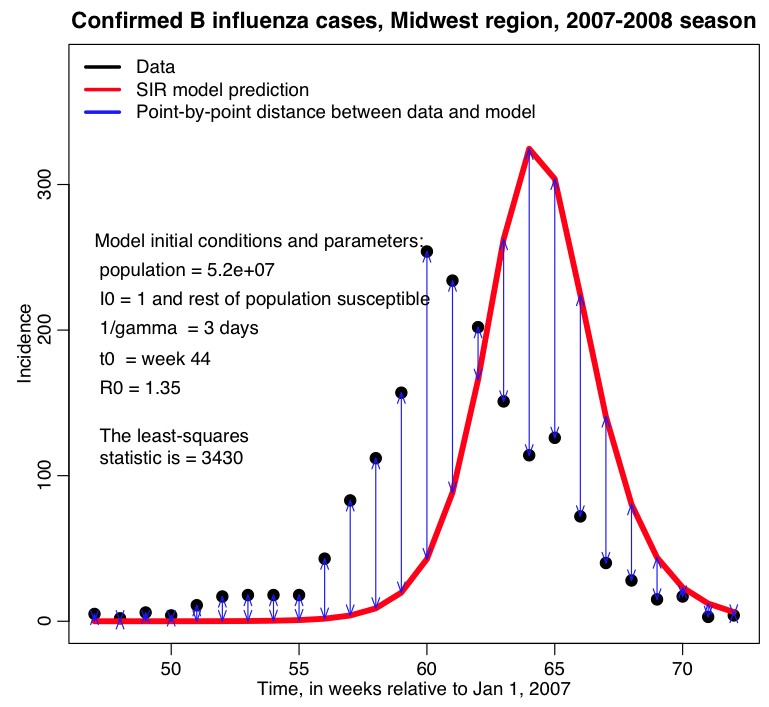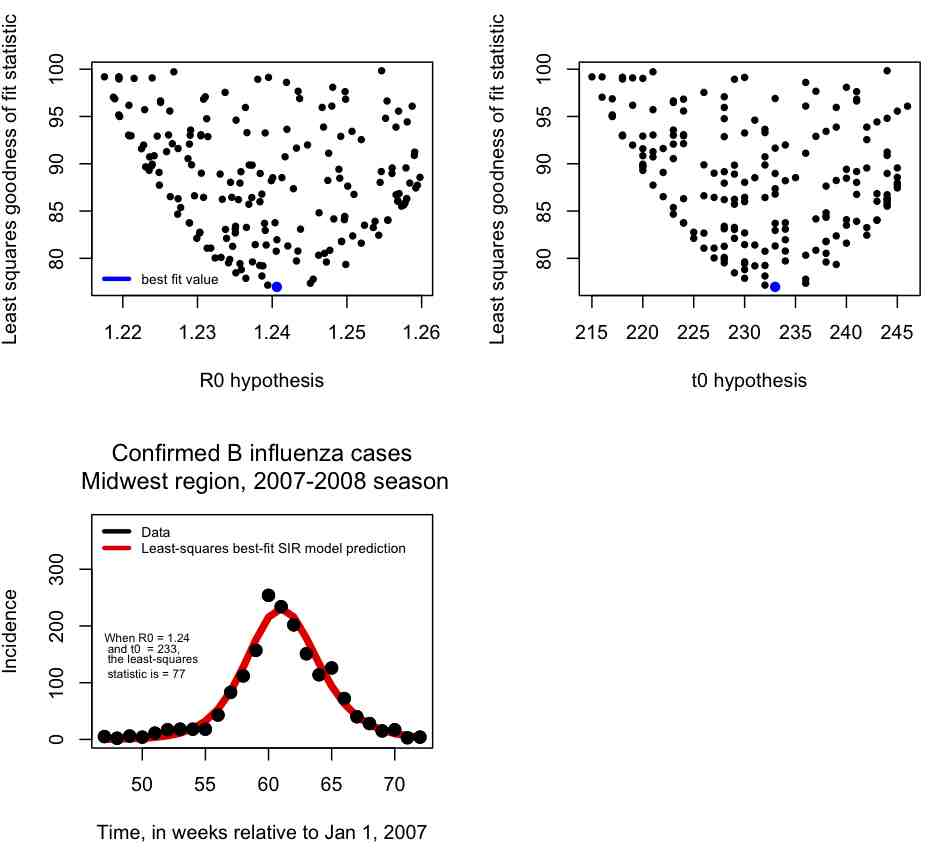# Another example C++ program to fit model parameters to data

In a past module, we examined how we could use methods in the R deSolve to fit the parameters of an SIR model to confirmed cases of influenza B in the Midwest region during the 2007-2008 flu season (the data were obtained from the CDC).  In that module, we used a Least Squares goodness-of-fit estimator.

Refresher: the old R script for doing the fit with parameter sweeps

The comma delimited data file I prepared that contains CDC influenza data from the Midwest for the 2007-2008 season, is in midwest_influenza_2007_to_2008.dat

In the fit_example.R script I get the estimated incidence from an SIR model, with population size equal to the population of the Midwest, and assuming that one infected person is introduced into a completely susceptible population at time t0 (is this a good assumption?).  The script assumes that t0 is week 44 of the year, and that the R0 of the epidemic is 1.35.  I assume that the infectious period for flu is 1/gamma=3 days. In this example we assume that there is just one infected person at time t0, and that the population was fully immune.  In reality, those initial conditions are also unknown model parameters that need to be estimated or the assumptions clearly stated.

The script produces the following plot:The values of R0 and t0 that I used in that script are clearly not ideal!

Rather than changing R0 and t0, and re-running the fit_example.R script many different times, it is much easier to write an R script to do a loop where it randomly samples many different hypotheses for R0 and t0 and calculates the least-squares statistic, keeping track of how the least squares statistic depends on R0 and t0.In blue I show the value of (R0,t0) that minimizes the Least Squares statistic, and then in the lower plot I overlay the best fit curve on the data.  The fit looks quite reasonable.

In the file  fit_iteration.R I provide the code that (eventually, after running a while) produces the above plots.  Note that when you try this code out it will run slowly.  This is because many, many different combinations of R0 and t0 had to be tried to get a good number of points in the plots, and a reasonable idea of what R0, t0 values will provide the best fit.  The more parameters you fit for, the more parameter combinations you will have to try to populate plots like the ones above.  In fact, the complexity of the process rises exponentially in the number of parameters.

A more sophisticated way: doing the parameter sweeps in C++ with utilities in the UsefulUtils and cpp_deSolve classes

A much faster way to do these fits is to write a C++ program to randomly sample parameter hypotheses, numerically solve the model under those hypotheses, and then calculate a goodness-of-fit statistic comparing the model to the data (this is especially fast if you have access to super-computing resources!)

In this past module, I introduced you to a C++ class, cpp_deSolve.h and cpp_deSolve.cpp, that I wrote that has a method SimulateModel to numerically solve systems of differential equations using the 4th order Runge-Kutta method.  The class has hard-coded derivative equations for the SIR, SEIR, and harmonic SIR models; if you want a different model, you will have to code up the derivative equations yourself in the ModelEquations method.

Example of using the cpp_deSolve class to numerically solve an SIR model

In the file SIR_example.cpp, I give an example of an implementation of this class to numerically solve an SIR model.  To compile and link the files, you can use the makefile makefile_solve by typing

`make -f makefile_solve SIR_example`

The program writes to standard output the temporal estimates of S and I and R. To run the file, and re-direct the results to a file called sir.out, type

`./SIR_example > sir.out`